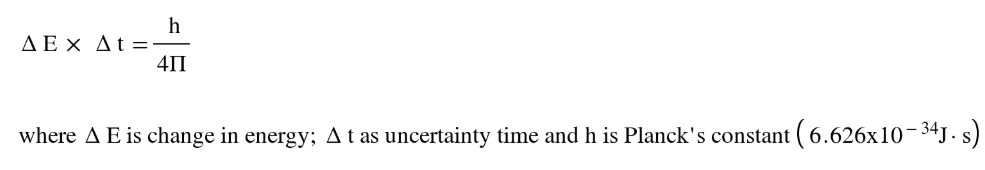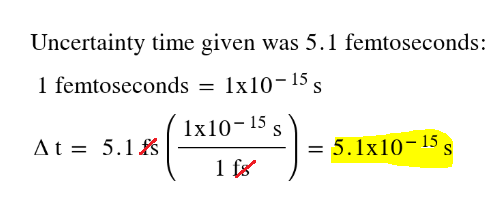# Problem: The 2005 Nobel Prize in Physics was given, in part, to scientists who had made ultrashort pulses of light. These pulses are important in making measurements involving very short time periods. One challenge in making such pulses is the uncertainty principle, which can be stated with respect to energy and time as ΔE • Δt ≥ h/4πlarge{Delta EcdotDelta t ge frac{ m h}{4pi}}. What is the energy uncertainty (ΔE) associated with a short pulse of laser light that lasts for only 5.1 femtoseconds (fs)? Suppose the low-energy end of the pulse had a wavelength of 713 nm.

###### FREE Expert Solution

Uncertainty principle can be solved using energy and time as:Calculate E from the Uncertainty principle equation using the given time:Plugging in this to the UP equation, find the ΔE:###### Problem Details

The 2005 Nobel Prize in Physics was given, in part, to scientists who had made ultrashort pulses of light. These pulses are important in making measurements involving very short time periods. One challenge in making such pulses is the uncertainty principle, which can be stated with respect to energy and time as ΔE • Δt ≥ h/4π. What is the energy uncertainty (ΔE) associated with a short pulse of laser light that lasts for only 5.1 femtoseconds (fs)? Suppose the low-energy end of the pulse had a wavelength of 713 nm.